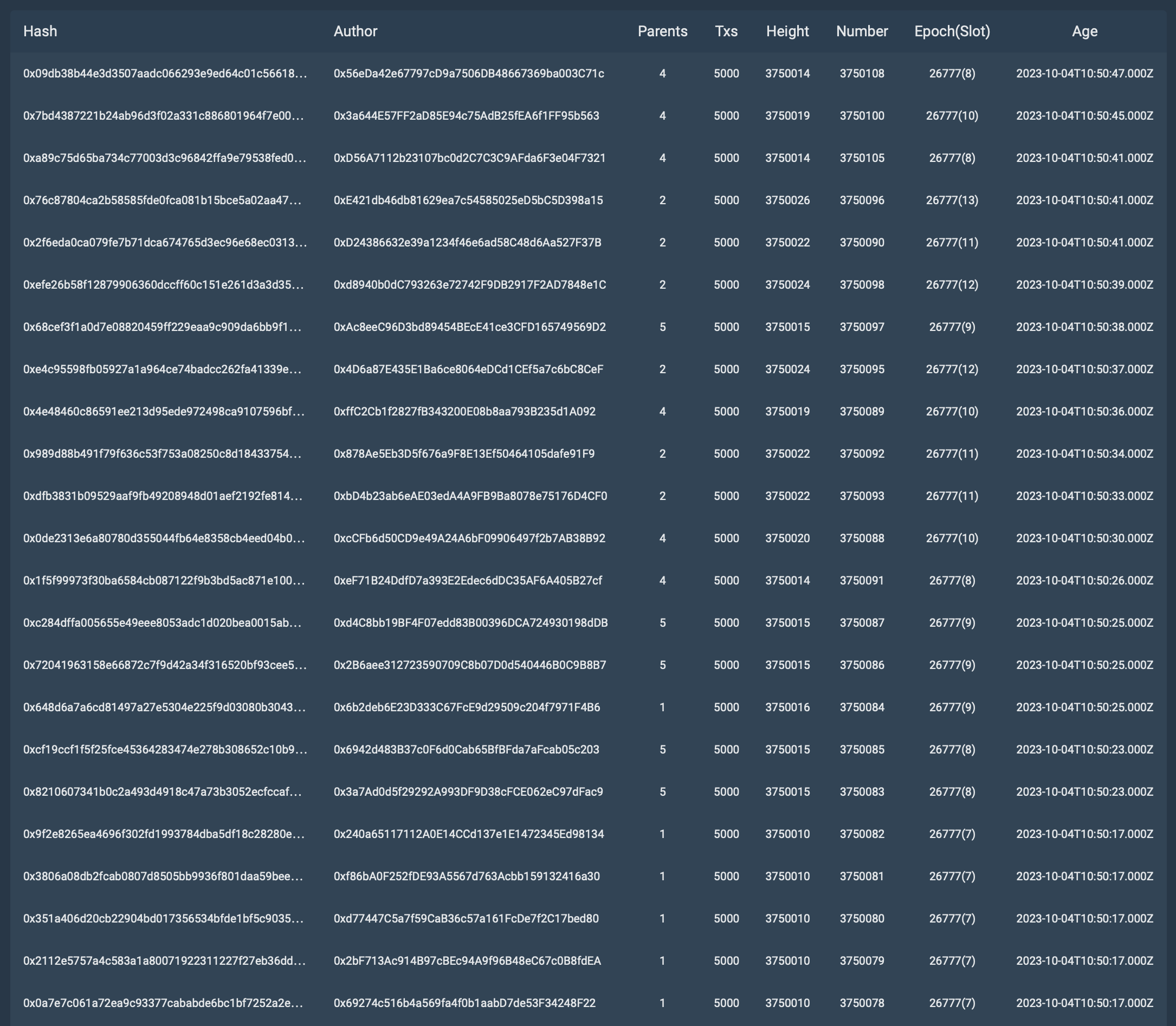# Testnet 7 Test 12

For this test, we used Digital Ocean Basic Droplet with the following server characteristics:

• Name: `s-2vcpu-4gb`
• vCPUs: `2`
• Memory (GiB): `4.0`
• SSD Disk (GiB): `80.0`
• Network Transfer (TB): `4.0`

Network settings:

• Slot duration: `4 sec`
• Nodes: `64`
• Workers: `32 per node`

Algorithm of actions during testing:

1. Stop creating blocks in the tested network.
2. Generates ~120,000 transactions.
3. Start 64 nodes.
4. Fix the time in which all these transactions were added and accepted into blocks, i.e. executed.

Let’s analyze the line from the test results below:

Block number: 3750078
Number of transactions: 5000
Age(slot): 26777(7)

Block number: 3750079
Number of transactions: 5000
Age(slot): 26777(7)

Block number: 3750080
Number of transactions: 5000
Age(slot): 26777(7)

Block number: 3750081
Number of transactions: 5000
Age(slot): 26777(7)

Block number: 3750082
Number of transactions: 5000
Age(slot): 26777(7)

Block number: 3750083
Number of transactions: 5000
Age(slot): 26777(8)

Block number: 3750085
Number of transactions: 5000
Age(slot): 26777(8)

Block number: 3750091
Number of transactions: 5000
Age(slot): 26777(8)

Block number: 3750105
Number of transactions: 5000
Age(slot): 26777(8)

Block number: 3750108
Number of transactions: 5000
Age(slot): 26777(8)

Block number: 3750084 Number of transactions: 5000
Age(slot): 26777(9)

Block number: 3750086 Number of transactions: 5000
Age(slot): 26777(9)

Block number: 3750087 Number of transactions: 5000
Age(slot): 26777(9)

Block number: 3750097 Number of transactions: 5000
Age(slot): 26777(9)

Block number: 3750088 Number of transactions: 5000
Age(slot): 26777(10)

Block number: 3750089 Number of transactions: 5000
Age(slot): 26777(10)

Block number: 3750100 Number of transactions: 5000
Age(slot): 26777(10)

Block number: 3750090 Number of transactions: 5000
Age(slot): 26777(11)

Block number: 3750092 Number of transactions: 5000
Age(slot): 26777(11)

Block number: 3750093 Number of transactions: 5000
Age(slot): 26777(11)

Block number: 3750095 Number of transactions: 5000
Age(slot): 26777(12)

Block number: 3750098 Number of transactions: 5000
Age(slot): 26777(12)

Let’s check how many transactions were added and processed in the two slots.
Take 5 slots = 20 seconds.
26777(7)-26777(11)

(5000+5000+5000+5000+5000 + 5000+5000+5000+5000+5000 + 5000+5000+5000+5000 + 5000+5000+5000 + 5000+5000+5000) = 100000

100000/20=5000 TPS.

Now let’s check the values for five slots.
If we take the 5th slot (26777(12)).
(100000 + 5000+5000) = 110000

110000/24=4583 TPS.